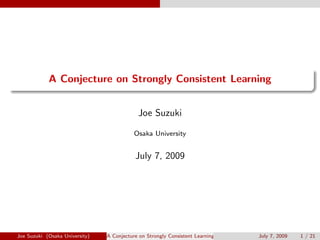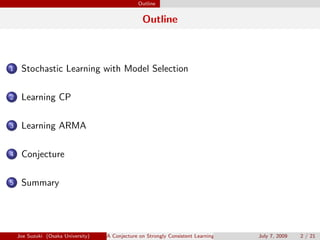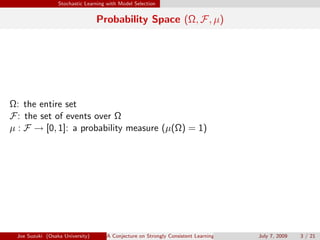Successfully reported this slideshow.

# A Conjecture on Strongly Consistent Learning

0

Share×
1 of 21
1 of 21

# A Conjecture on Strongly Consistent Learning

0

Share

A Conjecture on Strongly Consistent Learning
Joe Suzuki, Osaka University, July 7, 2009

A Conjecture on Strongly Consistent Learning
Joe Suzuki, Osaka University, July 7, 2009

## More Related Content

### Related Books

Free with a 14 day trial from Scribd

See all

### Related Audiobooks

Free with a 14 day trial from Scribd

See all

### A Conjecture on Strongly Consistent Learning

1. 1. . . A Conjecture on Strongly Consistent Learning Joe Suzuki Osaka University July 7, 2009 Joe Suzuki (Osaka University) A Conjecture on Strongly Consistent Learning July 7, 2009 1 / 21
2. 2. Outline Outline 1 Stochastic Learning with Model Selection 2 Learning CP 3 Learning ARMA 4 Conjecture 5 Summary Joe Suzuki (Osaka University) A Conjecture on Strongly Consistent Learning July 7, 2009 2 / 21
3. 3. Stochastic Learning with Model Selection Probability Space (Ω, F, µ) Ω: the entire set F: the set of events over Ω µ : F → [0, 1]: a probability measure (µ(Ω) = 1) Joe Suzuki (Osaka University) A Conjecture on Strongly Consistent Learning July 7, 2009 3 / 21
4. 4. Stochastic Learning with Model Selection Stochastic Learning X : Ω → R: random variable µX : the measure w.r.t. X x1, · · · , xn ∈ X(Ω): n samples   Induction/Inference . µX −→ x1, · · · , xn (Random Number Generation) x1, · · · , xn −→ µX (Stochastic Learning) Joe Suzuki (Osaka University) A Conjecture on Strongly Consistent Learning July 7, 2009 4 / 21
5. 5. Stochastic Learning with Model Selection Two Problems with Model Selection Conditional Probability for Y given X . . µY |X Identify the equivalence relation in X from samples. x ∼ x′ ⇐⇒ µ(Y = y|X = x) = µ(Y = y|X = x′ ) for y ∈ Y (Ω) ARMA for {Xn}∞ n=−∞ . Xn + ∑k j=1 λj Xn−j ∼ N(0, σ2) with {λ}k j=1 (0 ≤ k < ∞) Identify the true order k from samples. This paper compares CP and ARMA to ﬁnd how close they are. Joe Suzuki (Osaka University) A Conjecture on Strongly Consistent Learning July 7, 2009 5 / 21
6. 6. Learning CP CP A ∈ F G ⊆ F CP of A given G (Radon-Nykodim) . . ∃G-measurable g : Ω → R s.t µ(A ∩ G) = ∫ G g(ω)µ(dω) for G ∈ G. CP µY |X A := (Y = y), y ∈ Y (Ω) G: generated by subsets of X. Assumption |Y (Ω)| < ∞ Joe Suzuki (Osaka University) A Conjecture on Strongly Consistent Learning July 7, 2009 6 / 21
7. 7. Learning CP Applications for CP Stochastic Decision Trees, Stochastic Horn Clauseses Y |X, etc. . . Find G from samples. G = {G1, G2, G3, G4, G5} "! # "! # "! # "! # "! # "! # "! # "! # ¡ ¡¡ e ee ¡ ¡¡ e ee ¨¨¨¨¨¨ rrrrrr Weather Temp G3 Wind G1 G2 G4 G5 Clear Cloud Rain ≤ 75 > 75 Yes No Joe Suzuki (Osaka University) A Conjecture on Strongly Consistent Learning July 7, 2009 7 / 21
8. 8. Learning CP Applications for CP (cont’d) Finite Bayesian Networks Xi |Xj , j ∈ π(i) . . Find π(i) ⊆ {1, · · · , i − 1} from samples. X3 X4 X5 X2 X1 T E E d d ds      © d d d Joe Suzuki (Osaka University) A Conjecture on Strongly Consistent Learning July 7, 2009 8 / 21
9. 9. Learning CP Learning CP zi := (xi , yi ) ∈ Z(Ω) := X(Ω) × Y (Ω) From zn := (z1, · · · , zn) ∈ Zn(Ω), ﬁnd a minimal G. G∗: true ˆGn: estimated Assumption . . |G∗| ∞ Two Types of Errors G∗ ⊂ ˆGn (Over Estimation) G∗ ̸⊆ ˆGn (Under Estimation) Joe Suzuki (Osaka University) A Conjecture on Strongly Consistent Learning July 7, 2009 9 / 21
10. 10. Learning CP Example: Quinlan’s Q4.5 (a) G∗ ¡¡ ee ¡¡ ee ¨¨¨¨ rrrr Weather Temp 3 Wind 1 2 4 5 Clear Cloud Rain ≤ 75 75 Yes No (b) Overestimation ¡¡ ee ¡¡ ee ¨¨¨¨ rrrr Weather Temp Wind Wind 1 2 5 6 3 4 Clear Cloud Rain ≤ 75 75 Yes No Yes No ¡¡ ee (c) Underestimation ¡¡ ee ¨¨¨¨ rrrr Weather Temp 3 4 1 2 Clear Cloud Rain ≤ 75 75 (d) Underestimation ¨¨¨¨ rrrr Weather 1 Wind Wind 4 5 ¡¡ ee ¡¡ ee 2 3 Clear Cloud Rain Yes No ¡¡ ee Joe Suzuki (Osaka University) A Conjecture on Strongly Consistent Learning July 7, 2009 10 / 21
11. 11. Learning CP Information Criteria for CP Given zn ∈ Z(Ω), ﬁnd G minimizing I(G, zn ) := H(G, zn ) + k(G) 2 dn H(G, zn): Empirical Entropy (Fitness of zn to G) k(G): the # of Parameters (Simplicity of G) dn ≥ 0: dn n → 0 dn = log n BIC/MDL dn = 2 AIC Joe Suzuki (Osaka University) A Conjecture on Strongly Consistent Learning July 7, 2009 11 / 21
12. 12. Learning CP Consistency Consistency ( ˆGn −→ G∗ (n → ∞)) . . Weak Consistency Probability Convergence (O(1) dn o(n)) Strong Consistency Almost Surely Convergence (MDL/BIC etc.) AIC (dn = 2) is not consistent because {dn} is too small ! Problem What is the minimum {dn} for Strong Consistency ? Answer (Suzuki, 2006) dn = 2 log log n (the Law of Iterated Logarithms) Joe Suzuki (Osaka University) A Conjecture on Strongly Consistent Learning July 7, 2009 12 / 21
13. 13. Learning CP Error Probability for CP G∗ ⊂ G (Over Estimation) . . µ{ω ∈ Ω|I(G, Zn (ω)) I(G∗ , Zn (ω))} = µ{ω ∈ Ω|χ2 K(G)−K(G∗)(ω) (K(G) − K(G∗ ))dn} χ2 K(G)−K(G∗) ∼ χ2 of freedom K(G) − K(G∗) G∗ ̸⊆ G (Under Estimation) µ{ω ∈ Ω|I(G, Zn (ω)) I(G∗ , Zn (ω)) diminishes exponentially with n Joe Suzuki (Osaka University) A Conjecture on Strongly Consistent Learning July 7, 2009 13 / 21
14. 14. Learning CP Applications for ARMA Time Series Xi |Xi−k, · · · , Xi−1 . . Xi + k∑ j=1 λj Xi−j ∼ N(0, σ2 ) Find k from samples. Gaussian Bayesian Networks Xi |Xj , j ∈ π(i) Xi + ∑ j∈π(i) λj,i Xj ∼ N(0, σ2 i ) Find π(i) ⊆ {1, · · · , i − 1} from samples. Joe Suzuki (Osaka University) A Conjecture on Strongly Consistent Learning July 7, 2009 14 / 21
15. 15. Learning ARMA Learning ARMA k ≥ 0 {λj }k j=1: λi ∈ R σ2 ∈ R0 {Xi }∞ i=−∞: Xi + k∑ j=1 λj Xi−j = ϵi ∼ N(0, σ2 ) Given xn := (x1, · · · , xn) ∈ X1(Ω) × · · · × Xn(Ω), estimate k: known {λj }k j=1, σ2 k: Unknown k as well as {λj }k j=1, σ2 Joe Suzuki (Osaka University) A Conjecture on Strongly Consistent Learning July 7, 2009 15 / 21
16. 16. Learning ARMA Yule-Walker If k is known, solve {ˆλj,k}k j=1 and ˆσ2 k w.r.t. ¯x := 1 n n∑ i=1 xi cj := 1 n n−j∑ i=1 (xi − ¯x)(xi+j − ¯x) , j = 0, · · · , k        −1 c1 c2 · · · ck 0 c0 c1 · · · ck−1 0 c1 c0 · · · ck−2 ... ... ... ... ... 0 ck−1 ck−2 · · · c0               ˆσ2 k ˆλ1,k ˆλ2,k ... ˆλk,k        =        −c0 −c1 −c2 ... −ck        Joe Suzuki (Osaka University) A Conjecture on Strongly Consistent Learning July 7, 2009 16 / 21
17. 17. Learning ARMA Information Criteria for ARMA If k is unknown, given xn ∈ Xn(Ω), ﬁnd k minimiziing I(k, xn ) := 1 2 log ˆσ2 k + k 2 dn ˆσ2 k: obtain using Yule-Walker dn ≥ 0: dn n → 0 dn = log n BIC/MDL dn = 2 AIC dn = 2 log log n Hannan-Quinn (1979) =⇒the minimum {dn} satisfying Strong Consistency Suzuki (2006) was inspired by Hannan-Quinn (1979) ! Joe Suzuki (Osaka University) A Conjecture on Strongly Consistent Learning July 7, 2009 17 / 21
18. 18. Learning ARMA Error Probability for ARMA k∗: true Order k∗ k (Under Estimation) . µ{ω ∈ Ω|I(k, Xn (ω)) I(k∗, Xn (ω))} diminishes exponentially with n Joe Suzuki (Osaka University) A Conjecture on Strongly Consistent Learning July 7, 2009 18 / 21
19. 19. Conjecture Error Probability for ARMA (cont’d) Conjecture: k∗ k (Over Estimation) . µ{ω ∈ Ω|I(k, Xn (ω)) I(k∗, Xn (ω))} = µ{ω ∈ Ω|χ2 k−k∗ (ω) (k − k∗)dn} χ2 k−k∗ ∼ χ2 of freedom k − k∗ Joe Suzuki (Osaka University) A Conjecture on Strongly Consistent Learning July 7, 2009 19 / 21
20. 20. Conjecture In fact, ... For k k∗ and large n (use ˆσ2 k = (1 − ˆλ2 k,k)σ2 k−1), 1 2 log ˆσ2 k + k 2 dn k∗ 2 log σ2 k∗ + k∗ 2 dn ⇐⇒ k∑ j=k∗+1 log ˆσ2 j ˆσ2 j−1 (k − k∗)dn ⇐⇒ k∑ j=k∗+1 log(1 − ˆλ2 k,k) dn ⇐⇒ k∑ j=k∗+1 ˆλ2 k,k (k − k∗)dn It is very likely that ∑k j=k∗+1 ˆλ2 k,k ∼ χ2 k−k∗ although ˆλk,k ̸∼ N(0, 1). Joe Suzuki (Osaka University) A Conjecture on Strongly Consistent Learning July 7, 2009 20 / 21
21. 21. Summary Summary CP and ARMA are similar . . 1 Results in ARMA can be applied to CP 2 Results in Gausssian BN can be applied to ﬁnite BN.   The conjecture is likely enough ? . . Answer: Perhaps. Several evidences. {Zi }n i=1: independent Zi ∼ N(0, 1) rankA = n − k, A2 = A =⇒ t [Z1, · · · , Zn]A[Z1, · · · , Zn] ∼ χ2 n−k Joe Suzuki (Osaka University) A Conjecture on Strongly Consistent Learning July 7, 2009 21 / 21